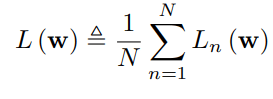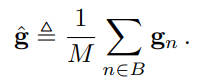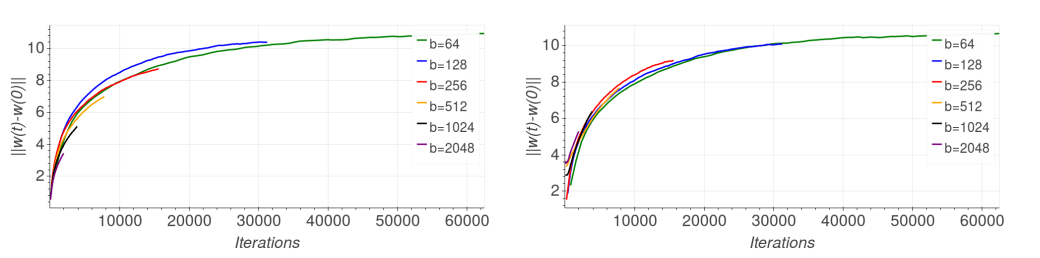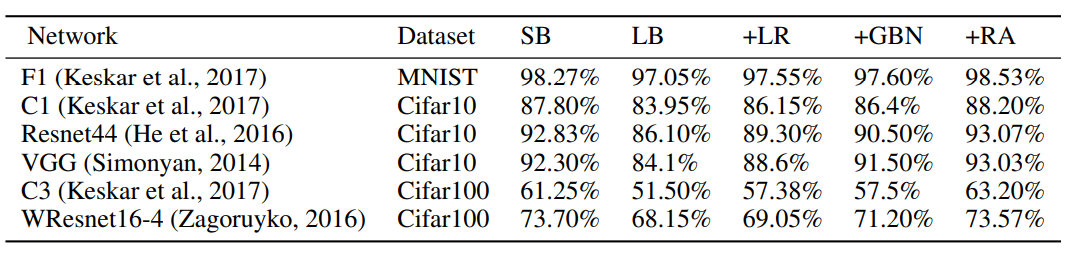# A Closer Look at the Generalization Gap in Large Batch Training of Neural Networks

This research addresses a well-known phenomenon regarding large batch sizes during training and the generalization gap.

## Introduction

Deep learning architectures such as recurrent neural networks and convolutional neural networks have seen many significant improvements and have been applied in the fields of computer vision, speech recognition, natural language processing, audio recognition and more. The most commonly used optimization method for training highly complex and non-convex DNNs is stochastic gradient descent (SGD) or some variant of it. DNNs however typically have some non-convex objective functions which are a bit difficult optimize with SGD. Thus, SGD, at best, finds a local minimum of this objective function. Although the solutions of DNNs are a local minima, they have produced great end results. The 2015 paper The Loss Surfaces of Multilayer Networks by Choromanska et al. showed that as the number of dimensions increases, the difference between local minima decreases significantly, and, therefore, the existence of “bad” local minima decreases exponentially.

In the 2018 Israel Institute of Technology paper we will primarily examine in this article, Train Longer, Generalize Better: Closing the Generalization Gap in Large Batch Training of Neural Networks, Hoffer et al. address a well-known phenomenon regarding large batch sizes during training and the generalization gap. That is, when a large batch size is used while training DNNs, the trained models appear to generalize less well. This observation remains true even when the models are trained “without any budget or limits, until the loss function ceased to improve” (Keskar et al., 2017).

The generalization gap is an important concept for understanding generalization. It is commonly defined as the difference between a model’s performance on training data and its performance on unseen data drawn from the same distribution. Significant strides have been made toward deriving better DNN generalization bounds. This is because understanding the origin of the generalization gap, and moreover, finding ways to decrease it, may have a significant practical importance.

Hoffer et al. study the above phenomenon in their paper. They examine the initial high learning rate training phase and propose that this phase can be described using a high-dimensional “random walk on a random potential” process, with an “ultra-slow” logarithmic increase in the distance of the weights from their initialization. The authors observed the following,

• by simply adjusting the learning rate and batch normalization, the generalization gap can be significantly decreased,
• generalization, what is the model’s ability to adapt properly to new, previously unseen data, keeps improving for a long time at the initial high learning rate, even without any observable changes in training or validation errors, and
• there is no inherent “generalization gap”, i.e., large-batch training can generalize as well as small-batch training by adapting the number of iterations.

Therefore, the authors concluded empirically that the “generalization gap” stems from the relatively small number of updates rather than the batch size itself, and can be completely eliminated by adapting the training regime used.

We will look at these findings in more detail in the rest of this article.

## Training with a large batch, background

Training method setup
Typically when training DNNs, the learning rate and momentum term are perturbed over time, usually with an exponential decrease every few epochs of training data. Another common approach is to use a “regime” adaptive parameter method. This is done by defining the regimes the optimization process is in. These regimes define intervals for which the learning rates are constant during the entirety of the regime.

Hoffer et al. examine a fixed learning rate (within a certain regime) that decreases exponentially every few epochs. Here the number of epochs is related to the regime the training process is in. As a side note, the convergence of SGD is known to be affected by the batch size (Li et al., 2014), but the authors here only focus on generalization. The experiments were carried out using the Resnet44 topology, introduced by He et al. (2016).

Empirical observations from previous work
Previous work by Keskar et al. (2017) studied the performance and properties of models trained with relatively large batches and reported the following observations:

• Training models with large batch sizes increased the generalization error.
• The “generalization gap” did not go away even when the models were trained without limits — that is, until the loss function stops improving.
• Low generalization was correlated with “sharp” minima (strong positive curvature), while good generalization was correlated with “flat” minima (weak positive curvature).
• Small-batch regimes were briefly noted to produce weights that are farther away from the initial point, in comparison with the weights produced in a large-batch regime.

## Theoretical analysis

Notation
This paper examines DNNs trained with SGD. Let N denote the number of samples which the DNN is trained on, w the vector of the neural network parameters, and L_n(w) the loss function on sample n. The value w is determined by minimizing the loss function L(w) as defined in Equation (1).Equation (1). Loss function to be optimized during training

SGD will compute the negative gradient of the loss function L(w) and use it as the descent direction. The gradient is shown in Equation (2).

where g is the true gradient, and g_n is the per-sample gradient. During training, we increment the parameter vector w using only the mean gradient gˆ computed on some mini-batch B, i.e., a set of M randomly selected sample indices as defined in Equation (3).Equation (3). The mean gradient per mini-batch. This is used to update w at each SGD iteration.

The authors examine the simplest form of SGD training, in which the weights at update step t are incremented according to the mini-batch gradient ∆w_t = η gˆ_t. In addition, the increments are uncorrelated between different mini-batches. Here is where the idea of a random walk comes into play. We can think of the weight vector w_t as a particle performing a random walk on the loss surface of L(w_t). Furthermore, adding the momentum term can be thought of as providing the particle with inertia.

Motivation of random walk model and empirical observations
The reason behind the use of a random walk intuition has to do with the fact that the shape/surface of the loss function in DNNs cannot be determined. Statistical models and tools are commonly used to formulate a simpler description of the loss function as a random process, and therefore, the motivation for using a random walk.

In addition, “random walk on a random potential (loss)” is a field that has been studied extensively. Bouchaud & Georges, in 1990, showed that the asymptotic behaviour of the auto-covariance of a random potential,

in a certain range, determines the asymptotic behaviour of the random walker in that range:

This is called an “ultra-slow diffusion” in which, typically || w_t – w_0 || ~ log(t)^(2/α). In other words, the mean square displacement grows logarithmically with time. From a training point of view, this behaviour tells us that the weight distance from the initialization point increases logarithmically with the number of training iterations (weight updates).

The authors found, empirically, that the value of α = 2. Moreover, the authors found that for all batch sizes, a very similar logarithmic graph is observed. However, different graphs for different batch sizes seem to have somewhat different slopes. This indicates a somewhat different diffusion rate for different batch sizes. Another observation was that smaller batch sizes entail more training iterations in total. Thus, there is a significant difference in the number of iterations and the corresponding weight distance reached at the end of the initial learning phase.

This leads to the following informal argument (which assumes flat minima are indeed important for generalization). During the initial training phase, to reach a minima of “width” d the weight vector w_t has to travel at least a distance d, and this takes a long time, which is about exp(d) iterations. Thus, to reach wide/flat minima we need to have the highest possible diffusion rates (which do not result in numerical instability) and a large number of training iterations.

These observations are what drove the paper’s contribution. It was previously thought that large batch-sizes would result in generalization gaps. However, these observations provide evidence that training with large batch can be done without suffering from performance degradation.

## Matching weight increment statistics for different mini-batch sizes

First, to correct the different diffusion rates observed for different batch sizes, the paper matches the statistics of the weight increments to that of a small batch size. It does so by increasing the learning rate by the square root of the mini-batch size. The reasoning behind this decision is to have the weight updates in SGD be proportional to the estimated gradient, i.e., ∆w ∝ ηg^, where η is the learning rate. Furthermore, the covariance matrix of the parameter update step ∆w is defined in Equation (4).

Thus, we can see that in order to make the coefficient η^2/M = 1 so that the covariance matrix of the weight update step remains constant for all mini-batch sizes, η must be chosen to be the square root of the mini-batch size M. By implementing this adaptive learning rate scheme, the results of ||w_t − w_0|| between the different batch sizes during the initial training phase show similar slopes. This is displayed in Figure 1.Figure 1. (left) Euclidean distance of weight vector from initialization before learning rate adjustment and GBN. (right) Euclidean distance of weight vector from initialization after learning rate adjustment and GBN.

A few things to note here is that by increasing the learning rate, the mean steps E[∆w] will also increase. However, the authors found that this effect is negligible since E[∆w] is typically orders of magnitude lower than the standard deviation. This is somewhat intuitive as the update steps in descent methods are generally very small perturbations rather than large changes, i.e., during each update step, the algorithm tweaks the weights by a small amount and evaluates the new value of the loss function.

Hoffer et al. also take into account the influence of batch normalization. Since each per-sample gradient g_n (Equation (3)) depends on the selected mini-batch. In addition, when working with batch-related gradient descent, the updates at the end of the training epoch require the additional complexity of accumulating prediction errors across all training examples. Hoffer et al. takes this into consideration and proposes a method called Ghost Batch Normalization to address this problem.

Ghost Batch Normalization
General Batch Normalization (BN) approaches in neural networks form perform normalization on their inputs and have a learnable mean and standard deviation. In the work on BN by Ioffe & Szegedy in 2015, the mean and variance are to be calculated for each channel or feature map separately across a mini-batch of data. For example, in a convolutional layer, the mean and variance are computed across all spatial locations and training examples in a mini-batch. Naturally, BN uses the batch statistics that depend on the chosen batch size.

Hoffer et al. propose theGhost Batch Normalization(GBN) method, which acquires the statistics on small virtual (“ghost”) batches instead of the real large batch. This has been observed to reduce the generalization error. In addition, the authors note that it is important to use the full batch statistic as suggested by Ioffe & Szegedy in 2015 for the inference phase. This is similar to the normal BN method where during inference, the statistics of each mini-batch are replaced with an exponential moving average of the mean and variance. This is to make inference behaviour independent of inference batch statistics.

The GBN algorithm is given in Algorithm 1 below.

It can be seen that the algorithm consists of calculating normalization statistics on disjoint subsets of each training batch. Specifically, with an overall batch size of B_L and a “ghost” batch size of B_S such that B_S evenly divides B_L, the normalization statistics are calculated as

The authors conclude that this form of batch norm update helps generalization and yields better results than computing the batch-norm statistics over the entire batch. In addition, they found that implementing both the adaptive learning rate and GBN adjustments improves generalization performance.

There is no clear explanation as to why GBN achieves this benefit, but some intuition can be reasoned. I think the following may provide an intuition into why it works: The methodology of GBN can be thought of as another form of regularization. That is, due to the stochasticity in normalization statistics caused by the random selection of mini-batches during training, Batch Normalization causes the representation of a training example to randomly change every time it appears in a different batch of data. Ghost Batch Normalization, by decreasing the number of examples that the normalization statistics are calculated over, increases the strength of this stochasticity, thereby increasing the amount of regularization.

## Adapting the number of weight updates eliminates generalization gap

Hoffer et al. stated that the initial training phase with a high-learning rate enables the model to reach farther locations in the parameter space, which may be necessary to find wider local minima and better generalization. As per Figure 1 (right)., the authors proceeded to match the graphs for different batch sizes by increasing the number of training iterations in the initial high-learning rate regime. They noticed that the distance between the current weight and the initialization point could be a good measure to determine when to decrease the learning rate.

Typically, learning rate adaptive algorithms decrease the learning rate after the validation error appears to reach a plateau. This practice is due to the long-held belief that the optimization process should not be allowed to decrease the training error when the validation error plateaus to avoid over-fitting. However, Hoffer et al. observed that substantial improvement to the final accuracy can be obtained by continuing the optimization using the same learning rate even if the training error decreases while the validation plateaus. Subsequent learning rate drops resulted, with a sharp validation error decrease and better generalization for the final model. This is displayed in Figure 2.Figure 2. Comparison of generalization error between large-batch regimes adapted to match performance of small-batch training. (left) validation error, (right) validation error zoomed in.

From these observations, Hoffer et al. concluded that the “generalization gap” phenomenon stems from the relatively small number of updates rather than the batch size. Thus, the authors adapted the training regime to better suit the usage of large mini-batches by modifying the number of epochs according to the mini-batch size used. This modification ensures that the number of optimization steps taken is identical to those performed in the small-batch regime and in turn, eliminates the generalization gap.

## Experimental results

To validate their findings, the authors experimented with the set of image classification tasks listed below.

• MNIST (LeCun et al., 1998b) – Consists of a training set of 60K and a test set of 10K 28 × 28 gray-scale images representing digits ranging from 0 to 9.
• CIFAR-10 and CIFAR-100 (Krizhevsky, 2009) – Each consists of a training set of size 50K and a test set of size 10K. Instances are 32 × 32 colour images representing 10 or 100 classes.
• ImageNet classification task Deng et al. (2009) – Consists of a training set of size 1.2M samples and test set of size 50K. Each instance is labelled with one of 1000 categories.

In each of the experiments, the authors used the training regime suggested by the original work, together with a momentum SGD optimizer. They defined a batch of 4096 samples as a large batch (LB) and a small batch (SB) was defined as either 128 or 256 samples. The authors then compared the original training baseline for small and large batches, as well as the methods proposed in this paper.

• Learning rate tuning (LB+LR): Using a large batch, while adapting the learning rate to be larger (than that of the small batch)
• Ghost batch norm (LB+LR+GBN): Additionally using the “Ghost batch normalization” method in the training procedure. The “ghost batch size” used is 128.
• Regime adaptation: Using the tuned learning rate as well as ghost batch-norm, but with an adapted training regime. The training regime is modified to have the same number of iterations for each batch size used — effectively multiplying the number of epochs by the relative size of the large batch.

The empirical results supported the paper’s main claim that there is no inherent generalization problem with training using large mini batches. This is shown in Table 1.Table 1. Validation accuracy results, SB/LB represent small and large batch respectively. GBN stands for Ghost-BN, and RA stands for regime adaptation

We can see that there is a visible generalization gap between using a small batch (SB) versus a large batch (LB). However, the methods proposed in this paper can significantly improve the validation accuracy to the point where the generalization gap completely disappears. Furthermore, in some cases the final validation accuracy is seen to be even better than the one obtained when using a small batch.

## Conclusion

The Hoffer et al. paper addresses one of the commonly known phenomena in training deep learning models: training with large batch size results in worse generalization compared to small batch sizes.

The paper modelled the “movement” on the loss surface as a random walk and studied the relationship of its diffusion rate to the size of a batch. This model provided the empirical observations which allowed Hoffer et al. to propose an adaptive learning rate scheme that depends on the batch size (the learning rate is chosen to be the square root of the mini-batch size). In addition, the authors proposed a novel Ghost Batch Normalization scheme which computes batch-norm statistics over several partitions (“ghost batch-norm”). Ghost Batch Normalization is beneficial for most batch sizes (bigger than the size of a “small batch”), has no computational overhead, is straightforward to tune, and can be potentially used in combination with inference example weighing to great effect. Finally, the authors proposed the use of a sufficient number of high learning rate training iterations.

The experiments carried out in this paper showed that it is indeed possible to enable training with large batches without suffering performance degradation, and that the generalization problem is not related to the batch size but rather to the amount of updates. This contribution re-examines the common belief that large batch sizes will result in poor generalization and provides methods for closing the generalization gap.

The paper Train Longer, Generalize Better: Closing the Generalization Gap in Large Batch Training of Neural Networks is on arXiv.

Analyst: Joshua Chou | Editor: H4O; Michael Sarazen

This report offers a look at how China has leveraged artificial intelligence technologies in the battle against COVID-19. It is also available on Amazon KindleAlong with this report, we also introduced a database covering additional 1428 artificial intelligence solutions from 12 pandemic scenarios.

Click here to find more reports from us.

We know you don’t want to miss any latest news or research breakthroughs. Subscribe to our popular newsletter Synced Global AI Weekly to get weekly AI updates.Machine Intelligence | Technology & Industry | Information & Analysis

## 13 comments on “A Closer Look at the Generalization Gap in Large Batch Training of Neural Networks”

1.360DigiTMG_vizag

Great post, I really loved it. Keep posting. Thanks for sharing.

proffessional courses

2.360DigiTMG 360

Set aside my effort to peruse all the remarks, however I truly delighted in the article. It’s consistently pleasant when you can not exclusively be educated, yet in addition, engaged!
data science course in noida

3.You really make it look so natural with your exhibition however I see this issue as really something which I figure I could never understand. It appears to be excessively entangled and incredibly expansive for me.
https://360digitmg.com/course/certification-program-in-data-science

4.360DigiTMG

The information you have posted is important. The objections you have insinuated was worthy. Thankful for sharing.
data science course in noida

5.I want to leave a little comment to support and wish you the best of luck.we wish you the best of luck in all your blogging enedevors.

6.Deekshitha

Informative blog
data analytics courses in hyderabad

7.suzi

Cookies are small blocks of data created by a web server while a user is browsing a website and placed on the user’s computer or other device by the user’s web browser. https://syncedreview.com/2020/09/13/a-closer-look-at-the-generalization-gap-in-large-batch-training-of-neural-networks/

8.Thanks for sharing this valuable information, we also provide instagram video download and,
really appreciate your hard work and good research that help us in for of your good valuable article. Keep updating us with your awesome content.

9.George Black

Training data is used by neural networks to learn and increase their accuracy over time. However, once these learning algorithms have been fine-tuned for accuracy, they become formidable tools in computer science and artificial intelligence, allowing us to classify and cluster data at rapid speeds. When compared to manual identification by human experts, tasks in speech recognition or picture recognition can be completed in minutes rather than hours. Google’s search algorithm is one of the most well-known neural networks. Artificial neural networks (ANNs) are made up of node layers, each of which has an input layer, one or more hidden layers, and an output layer. Save from TikTok

•use instagram reels download for best videos

10.Alba Jessica

Cyber security certification Course is an ideal possession for anyone pursuing this career.
Enroll for our Cyber Security Training Course Online

11.Mary

The information which you posted here is very helpful
Need Visa Service Visit Migration Agent Perth